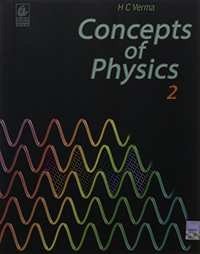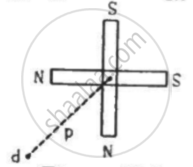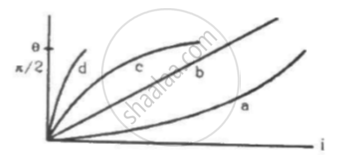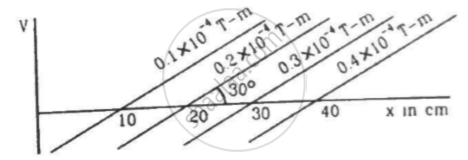Share

# HC Verma solutions for Class 12 Concepts of Physics Vol. 2 chapter 14 - Permanent Magnets [Latest edition]

Textbook page

#### Chapters## Chapter 14: Permanent Magnets

#### HC Verma solutions for Class 12 Concepts of Physics Vol. 2 Chapter 14 Permanent Magnets Exercise Short Answers [Pages 275 - 276]

Short Answers | Q 1 | Page 275

Can we have a single north pole, or a single south pole?

Short Answers | Q 2 | Page 275

Do two distinct poles actually exist at two nearby points in a magnetic dipole?

Short Answers | Q 3 | Page 275

An iron needle is attracted to the ends of a bar magnet but not to the middle region of the magnet. Is the material making up the ends of a bare magnet different from that of the middle  region?

Short Answers | Q 4 | Page 275

Compare the direction of the magnetic field inside a solenoid with that of the field there if the solenoid is replaced by its equivalent combination of north pole and south pole.

Short Answers | Q 5 | Page 275

Sketch the magnetic field lines for a current-carrying circular loop near its centre. Replace the loop by an equivalent magnetic dipole and sketch the magnetic field lines near the centre of the dipole. Identify the difference.

Short Answers | Q 6 | Page 275

The force on a north pole, vecF = mvecB , parallel to the field vecB . Does it contradict our earlier knowledge that a magnetic field can exert forces only perpendicular to itself?

Short Answers | Q 7 | Page 275

Two bar magnets are placed close to each other with their opposite poles facing each other. In absence of other forces, the magnets are pulled towards each other and their kinetic energy increases. Does it contradict our earlier knowledge that magnetic forces cannot do any work and hence cannot increase kinetic energy of a system?

Short Answers | Q 8 | Page 275

Magnetic scalar potential is defined as U(vec r_2) - U(vec r_1) = - ∫_vec(r_1)^vec(r_2) vec (B) . dvec(l)

Apply this equation to a closed curve enclosing a long straight wire. The RHS of the above equation is then -u_0 i by Ampere's law. We see that U(vec(r_2)) ≠ U(vec(r_1)) even when vec r_2 =vec r_1 .Can we have a magnetic scalar potential in this case?

Short Answers | Q 9 | Page 275

Can the earth's magnetic field be vertical at a place? What will happen to a freely suspended magnet at such a place? What is the value of dip here?

Short Answers | Q 10 | Page 276

Can the dip at a place be (a) zero (b) 90°?

Short Answers | Q 11 | Page 276

The reduction factor K of a tangent galvanometer is written on the instrument. The manual says that the current is obtained by multiplying this factor to tan θ. The procedure works well at Bhuwaneshwar. Will the procedure work if the instrument is taken to Nepal? If there is same error, can it be corrected by correcting the manual or the instrument will have to be taken back to the factory?

#### HC Verma solutions for Class 12 Concepts of Physics Vol. 2 Chapter 14 Permanent Magnets Exercise MCQ [Page 276]

MCQ | Q 1 | Page 276

A circular loop carrying a current is replaced by an equivalent magnetic dipole. A point on the axis of the loop is in

• end-on position

• both

• none

MCQ | Q 2 | Page 276

A circular loop carrying a current is replaced by an equivalent magnetic dipole. A point on the loop is in

• end-on position

• both

• none

MCQ | Q 3 | Page 276

When a current in a circular loop is equivalently replaced by a magnetic dipole,

• the pole strength m of each pole is fixed

• the distance d between the poles is fixed

• the product md is fixed

• none of the above

MCQ | Q 4 | Page 276

Let r be the distance of a point on the axis of a bar magnet from its centre. The magnetic field at such a point is proportional to

• 1/r

• 1/r^2

• 1/r^3

• none of these

MCQ | Q 5 | Page 276

Let r be the distance of a point on the axis of a magnetic dipole from its centre. The magnetic field at such a point is proportional to

• 1/r

• 1/r^2

• 1/r^3

• none of these

MCQ | Q 6 | Page 276

Two short magnets of equal dipole moments M are fastened perpendicularly at their centre in the Figure . The magnitude of the magnetic field at a distance d from the centre on the bisector of the right angle is• (u_0)/(4pi) M/d^3

• (u_0)/(4pi) (sqrt2M)/d^3

• (u_0)/(4pi) (2sqrt2M)/d^3

• (u_0)/(4pi) (2M)/d^3

MCQ | Q 7 | Page 276

Magnetic meridian is

• a point

• a line along north-south

• a horizontal plane

• a vertical plane

MCQ | Q 8 | Page 276

A compass needle which is allowed to move in a horizontal plane is taken to a geomagnetic pole. It

• will stay in north-south direction only

• will stay in east-west direction only

• will become rigid showing no movement

• will stay in any position

MCQ | Q 9 | Page 276

A dip circle is taken to geomagnetic equator. The needle is allowed to move in a vertical plane perpendicular to the magnetic meridian. The needle will stay

• in horizontal direction only

• in vertical direction only

• in any direction except vertical and horizontal

• in the direction it is released

MCQ | Q 10 | Page 276

Which of the following four graphs may best represent the current-deflection relation in a tangent galvanometer?MCQ | Q 11 | Page 276

A tangent galvanometer is connected directly to an ideal battery. If the number of turns in the coil is doubled the deflection will

• increase

• decrease

• remain unchanged

• either increase or decrease

MCQ | Q 12 | Page 276

If the current is doubled, the deflection is also doubled in

• a tangent galvanometer

• a moving-coil galvanometer

• both

• none

MCQ | Q 13 | Page 276

A very long bar magnet is placed with its north pole coinciding with the centre of a circular loop carrying as electric current i. The magnetic field due to the magnet at a point on the periphery of the wire is B. The radius of the loop is a. The force on the wire is

• very nearly 2πaiB perpendicular to the plane of the wire

• aiB in the plane of the wire

• πaiB along the magnet

• zero

#### HC Verma solutions for Class 12 Concepts of Physics Vol. 2 Chapter 14 Permanent Magnets Exercise MCQ [Page 277]

MCQ | Q 1 | Page 277

Pick the correct options.

(a) Magnetic field is produced by electric charges only
(b) Magnetic poles are only mathematical assumptions having no real existence
(b) A north pole is equivalent to a clockwise current and a south pole is equivalent to an anticlockwise current.
(d) A bar magnet is equivalent to a long, straight current.

MCQ | Q 2 | Page 277

A horizontal circular loop carries a current that looks clockwise when viewed from above. It is replaced by an equivalent magnetic dipole consisting of a south pole S and a north pole N.

(a) The line SN should be along a diameter of the loop.
(b) The line SN should be perpendicular to the plane of the loop
(c) The south pole should be slow the loop
(d) The north pole should be below the loop

MCQ | Q 3 | Page 277

Consider a magnetic dipole kept in the north to south direction. Let P1, P2, Q1, Q2 be four points at the same distance from the dipole towards north, south, east and west of the dipole respectively. The directions of the magnetic field due to the dipole are the same at

(a) P1 and P2
(b) Q1 and Q2
(c) P1 and Q1
(d) P2 and Q2

MCQ | Q 4 | Page 277

Consider the situation of the previous problem. The directions of the magnetic field due to the dipole are opposite at

(a) P1 and P2
(b) Q1 and Q2
(c) P1 and Q1
(d) P2 and Q2

MCQ | Q 5 | Page 277

To measure the magnetic moment of a bar magnet, one may use

(a) a tangent galvanometer
(b) a deflection galvanometer if the earth's horizontal field is known
(c) an oscillation magnetometer if the earth's horizontal field is known
(d) both deflection and oscillation magnetometer if the earth's horizontal field is not known

#### HC Verma solutions for Class 12 Concepts of Physics Vol. 2 Chapter 14 Permanent Magnets [Pages 277 - 278]

Q 1 | Page 277

A long bar magnet has a pole strength of 10 Am. Find the magnetic field at a point on the axis of the magnet at a distance of 5 cm from the north pole of the magnet.

Q 2 | Page 277

Two long bare magnets are placed with their axes coinciding in such a way that the north pole of the first magnet is 2.0 cm from the south pole of the second. If both the magnets have a pole strength of 10 Am, find the force exerted by one magnet of the other.

Q 3 | Page 277

A uniform magnetic field of 0.20 xx 10^-3  "T"  exists in the space. Find the change in the magnetic scalar potential as one moves through 50 cm along the field.

Q 4 | Page 277

Figure shows some of the equipotential surfaces of the magnetic scalar potential. Find the magnetic field B at a point in the region.Q 5 | Page 277

The magnetic field at a point, 10 cm away from a magnetic dipole, is found to be 2.0 xx 10^-4  "T" . Find the magnetic moment of the dipole if the point is (a) in end-on position of the dipole and (b) in broadside-on position of the dipole.

Q 6 | Page 277

Show that the magnetic field at a point due to a magnetic dipole is perpendicular to the magnetic axis if the line joining the point with the centre of the dipole makes an angle of tan^-1(sqrt 2) with the magnetic axis

Q 7 | Page 277

A bar magnet has a length of 8 cm. The magnetic field at a point at a distance 3 cm from the centre in the broadside-on position is found to be 4 xx 10^-6  "T".Find the pole strength of the magnet.

Q 8 | Page 277

A magnetic dipole of magnetic moment 1.44  "A m"^2is placed horizontally with the north pole pointing towards north. Find the position of the neutral point if the horizontal component of the earth's magnetic field is 18 μT.

Q 9 | Page 277

A magnetic dipole of magnetic moment 0.72 A m2 is placed horizontally with the north pole pointing towards south. Find the position of the neutral point if the horizontal component of the earth's magnetic field is 18 μT.

Q 10 | Page 277

A magnetic dipole of magnetic moment 0.72sqrt(2) "Am"^2 is placed horizontally with the north pole pointing towards east. Find the position of the neutral point if the horizontal component of the earth's magnetic field is 18 μT.

Q 11 | Page 277

The magnetic moment of the assumed dipole at the earth's centre is 8.0 × 1022 A m2. Calculate the magnetic field B at the geomagnetic poles of the earth. Radius of the earth is 6400 km.

Q 12 | Page 277

If the earth's magnetic field has a magnitude 3.4 × 10−5 T at the magnetic equator of the earth, what would be its value at the earth's geomagnetic poles?

Q 13 | Page 277

The magnetic field due to the earth has a horizontal component of 26 μT at a place where the dip is 60°. Find the vertical component and the magnitude of the field.

Q 14 | Page 277

A magnetic needle is free to rotate in a vertical plane which makes an angle of 60° with the magnetic meridian. If the needle stays in a direction making an angle of tan^-1(2sqrt(3)) with the horizontal, what would be the dip at that place?

Q 15 | Page 278

The needle of a dip circle shows an apparent dip of 45° in a particular position and 53° when the circle is rotated through 90°. Find the true dip.

Q 16 | Page 278

A tangent galvanometer shows a deflection of 45° when 10 mA of current is passed through it. If the horizontal component of the earth's magnetic field is B_H = 3.6 xx 10^-5  "T" and radius of the coil is 10 cm, find the number of turns in the coil.

Q 17 | Page 278

A moving-coil galvanometer has a 50-turn coil of size 2 cm × 2 cm. It is suspended between the magnetic poles producing a magnetic field of 0.5 T. Find the torque on the coil due to the magnetic field when a current of 20 mA passes through it.

Q 18 | Page 278

A short magnet produces a deflection of 37° in a deflection magnetometer in Tan-A position when placed at a separation of 10 cm from the needle. Find the ratio of the magnetic moment of the magnet to the earth's horizontal magnetic field.

Q 19 | Page 278

The magnetometer of the previous problem is used with the same magnet in Tan-B position. Where should the magnet be placed to produce a 37° deflection of the needle?

Q 20 | Page 278

A deflection magnetometer is placed with its arms in north-south direction. How and where should a short magnet having M/BH = 40 A m2 T−1 be placed so that the needle can stay in any position?

Q 21 | Page 278

A bar magnet takes π/10 second the complete one oscillation in an oscillation magnetometer. The moment of inertia of the magnet about the axis of rotation is 1.2 × 10−4 kg m2 and the earth's horizontal magnetic field is 30 μT. Find the magnetic moment of the magnet.

Q 22 | Page 278

The combination of two bar magnets makes 10 oscillations per second in an oscillation magnetometer when like poles are tied together and 2 oscillations per second when unlike poles are tied together. Find the ratio of the magnetic moments of the magnets. Neglect any induced magnetism.

Q 23 | Page 278

A short magnet oscillates in an oscillation magnetometer with a time period of 0.10 s where the earth's horizontal magnetic field is 24 μT. A downward current of 18 A is established in a vertical wire placed 20 cm east of the magnet. Find the new time period.

Q 24 | Page 278

A bar magnet makes 40 oscillations per minute in an oscillation magnetometer. An identical magnet is demagnetized completely and is placed over the magnet in the magnetometer. Find the time taken for 40 oscillations by this combination. Neglect any induced magnetism.

Q 25 | Page 278

A short magnet makes 40 oscillations per minute when used in an oscillation magnetometer at a place where the earth's horizontal magnetic field is 25 μT. Another short magnet of magnetic moment 1.6 A m2 is placed 20 cm east of the oscillating magnet. Find the new frequency of oscillation if the magnet has its north pole (a) towards north and (b) towards south.

## Chapter 14: Permanent Magnets## HC Verma solutions for Class 12 Concepts of Physics Vol. 2 chapter 14 - Permanent Magnets

HC Verma solutions for Class 12 Concepts of Physics Vol. 2 chapter 14 (Permanent Magnets) include all questions with solution and detail explanation. This will clear students doubts about any question and improve application skills while preparing for board exams. The detailed, step-by-step solutions will help you understand the concepts better and clear your confusions, if any. Shaalaa.com has the CBSE Class 12 Concepts of Physics Vol. 2 solutions in a manner that help students grasp basic concepts better and faster.

Further, we at Shaalaa.com provide such solutions so that students can prepare for written exams. HC Verma textbook solutions can be a core help for self-study and acts as a perfect self-help guidance for students.

Concepts covered in Class 12 Concepts of Physics Vol. 2 chapter 14 Permanent Magnets are Magnetic Substances, Magnetic Dipole Moment of a Revolving Electron, Current Loop as a Magnetic Dipole and Its Magnetic Dipole Moment, Introduction of Magnetism, Magnetisation and Magnetic Intensity, Curie Law of Magnetism, Magnetism and Gauss’s Law, Hysteresis Loop, Permanent Magnets and Electromagnets, Magnetic Properties of Materials, The Earth’S Magnetism, The Bar Magnet, Torque on a Magnetic Dipole (Bar Magnet) in a Uniform Magnetic Field, Dipole in a Uniform External Field, Magnetic Field Intensity Due to a Magnetic Dipole (Bar Magnet) Perpendicular to Its Axis, Magnetic Field Intensity Due to a Magnetic Dipole (Bar Magnet) Along Its Axis.

Using HC Verma Class 12 solutions Permanent Magnets exercise by students are an easy way to prepare for the exams, as they involve solutions arranged chapter-wise also page wise. The questions involved in HC Verma Solutions are important questions that can be asked in the final exam. Maximum students of CBSE Class 12 prefer HC Verma Textbook Solutions to score more in exam.

Get the free view of chapter 14 Permanent Magnets Class 12 extra questions for Class 12 Concepts of Physics Vol. 2 and can use Shaalaa.com to keep it handy for your exam preparation